### Direct Methods: Cramer’s Rule

### The Method

Cramer’s rule is one of the early methods to solve systems of linear equations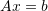. It is dated back to the eighteenth century! The method works really well; however, it is inefficient when the number of equations is very large. Nevertheless, with the current computing capabilities, the method can still be pretty fast. The method works using the following steps. First, the determinant of the matrix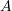is calculated. Each component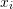of the unknowns can be computed as: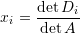where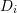is the matrix formed by replacing the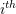column inwith the vector.

#### Example 1

Consider the linear system of equationsdefined as: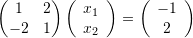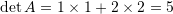. We will form the following two matrices by replacing the first column inwithand then the second column inwith: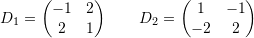The determinants of these matrices are: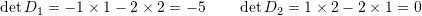Therefore: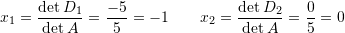The following is code that can be used for Cramer’s rule for a two-dimensional system of equations.

View Mathematica Code
A = {{a11, a12}, {a21, a22}};
AT = Transpose[A]
b = {b1, b2};
s1 = Drop[AT, {1}]
s2 = Drop[AT, {2}]
D1T = Insert[s1, b, 1]
D2T = Insert[s2, b, 2]
D1 = Transpose[D1T]
D2 = Transpose[D2T]
x1 = Det[D1]/Det[A]
x2 = Det[D2]/Det[A]

View Python Code
import sympy as sp
a11, a12, a21, a22 = sp.symbols("a11 a12 a21 a22")
A = sp.Matrix([[a11, a12], [a21, a22]])
print("A:",A)
AT = A.T
print("A Transpose:",AT)
s1 = sp.Matrix(AT)
s1.row_del(0)
print("s1:",s1)
s2 = sp.Matrix(AT)
s2.row_del(1)
print("s2:",s2)
b1, b2 = sp.symbols("b1 b2")
b = sp.Matrix([[b1, b2]])
D1T = sp.Matrix(s1)
D1T = D1T.row_insert(0,b)
print("D1T:",D1T)
D2T = sp.Matrix(s2)
D2T = D2T.row_insert(1,b)
print("D2T:",D2T)
D1 = D1T.T
print("D1T Transpose:",D1)
D2 = D2T.T
print("D2T Transpose:",D2)
x1 = D1.det()/A.det()
print("x1:",x1)
x2 = D2.det()/A.det()
print("x2:",x2)


#### Example 2

Consider the linear system of equationsdefined as: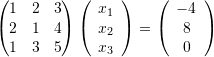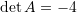. We will form the following three matrices by replacing the corresponding columns inwith: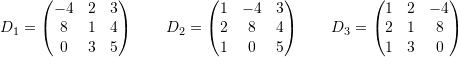The determinants of these matrices are: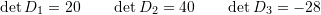Therefore: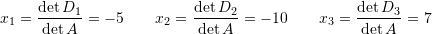The following procedure applies the Cramer’s rule to a general system. Test the procedure to see how fast it is for higher dimensions.

View Mathematica Code
Cramer[A_, b_] := (n = Length[A];
Di = Table[A, {i, 1, n}];
Do[Di[[i]][[All, i]] = b, {i, 1, n}];
x = Table[Det[Di[[i]]]/Det[A], {i, 1, n}])

A = {{1, 2, 3, 5}, {2, 0, 1, 4}, {1, 2, 2, 5}, {4, 3, 2, 2}};
b = {-4, 8, 0, 10};
Cramer[A, b]

View Python Code
import numpy as np
def Cramer(A, b):
n = len(A)
Di = [np.array(A) for i in range(n)]
for i in range(n):
Di[i][:,i] = b
return [np.linalg.det(Di[i])/np.linalg.det(A) for i in range(n)]
A = [[1, 2, 3, 5], [2, 0, 1, 4], [1, 2, 2, 5], [4, 3, 2, 2]]
b = [-4, 8, 0, 10]
Cramer(A, b)


The following link provides the MATLAB code for implementing the Cramer’s rule.